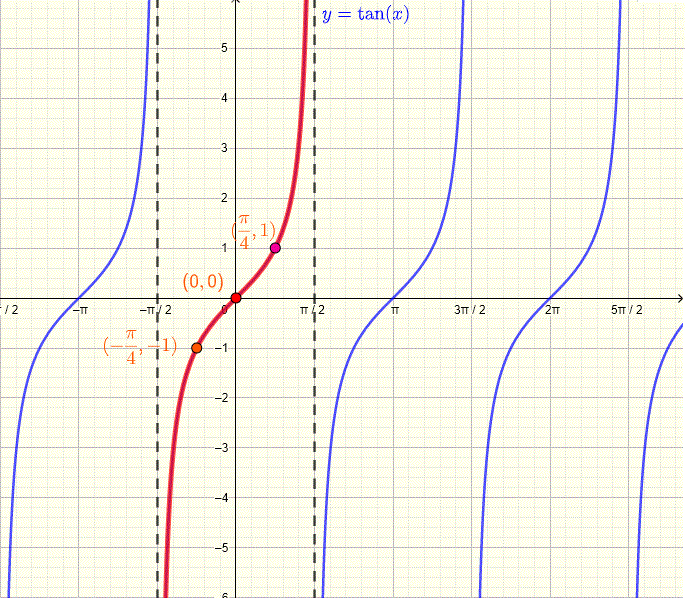# Tangent Function tan x

## Definition and Graph of the Tangent Function

We first consider angle $\theta$ with initial side on the positive x axis (in standard position) and terminal side OM as shown below.The tangent function is defined as
$\tan(\theta) = \dfrac{y}{x}$

From the definiton of the tangent, we can use the definitions of the $\sin(\theta)$ and $\cos(\theta)$ to deduce a relationship between tan, sin and cos functions as follows:
$\tan(\theta) = \dfrac{y}{x} = \dfrac{y/r}{x/r} = \dfrac{\sin(\theta)}{\cos(\theta)}$

Note that
1) $\tan(\theta+\pi) = \dfrac{\sin(\theta+\pi)}{\cos(\theta+\pi)} = \dfrac{-\sin(\theta)}{-\cos(\theta)}= \dfrac{\sin(\theta)}{\cos(\theta)} = \tan(\theta)$
and therefore $\tan(\theta)$ is a periodic function whose period is equal to $\pi$.

2) $\tan(-\theta) = \dfrac{\sin(-\theta)}{\cos(-\theta)} = \dfrac{-\sin(\theta)}{\cos(\theta)} = - \dfrac{\sin(\theta)}{\cos(\theta)} = - \tan(\theta)$
and therefore $\tan(\theta)$ is an odd function and its graph is symmetric with respect to the origin of a rectangular system of coordinates.

We now use a unit circle to find $\sin(\theta)$ and $\cos(\theta)$ and hence $\tan(\theta)$ over one period extending from $\theta = -\dfrac{\pi}{2}$ to $\theta = +\dfrac{\pi}{2}$.
We know from the sine and cosine functions that the x and y coordinates on a unit circle gives the values of $\sin(\theta)$ and $\cos(\theta)$ as shown below.Let us now put the values of the angles $-\dfrac{\pi}{2}, -\dfrac{\pi}{4} , 0 , \dfrac{\pi}{4} , \dfrac{\pi}{2}$ and the values of \cos(\theta) and \sin(\theta) on a table as shown below.

 $\theta$ $\cos(\theta)$ $\sin(\theta)$ $\tan(\theta) = \dfrac{\sin(\theta)}{\cos(\theta)}$ $-\dfrac{\pi}{2}$ $0$ $-1$ $undefined$ $-\dfrac{\pi}{4}$ $\dfrac{\sqrt 2}{2}$ $-\dfrac{\sqrt 2}{2}$ $-1$ $0$ $1$ $0$ $0$ $\dfrac{\pi}{4}$ $\dfrac{\sqrt 2}{2}$ $\dfrac{\sqrt 2}{2}$ $1$ $\dfrac{\pi}{2}$ $0$ $1$ $undefined$

$\tan(\theta)$ is undefined at $\theta = \dfrac{\pi}{2}$ and $\theta = - \dfrac{\pi}{2}$, however we can get information about the behaviour $\tan(\theta)$ close to these values
We use the calculator to find values of $\tan(\theta)$ as $\theta$ approaches $\dfrac{\pi}{2} \approx 1.570796327$ starting at $\theta = 1.500000$
 $\theta$ $\tan(\theta)$ $1.500000$ $14.10141995$ $1.550000$ $48.07848248$ $1.570000$ $1255.765592$ $1.570700$ $10381.32742$ $1.570791$ $187730.1491$ $1.570796$ $3060023.307$

As $\theta$ approaches $\dfrac{\pi}{2}$ by values smaller than $\dfrac{\pi}{2}$, $\tan(\theta)$ approaches large values and hence the existence of a vertical asymptote at $\theta = \dfrac{\pi}{2}$
Similarly, as $\theta$ approaches $- \dfrac{\pi}{2}$ by values larger than $- \dfrac{\pi}{2}$ , $\tan(\theta)$ approaches small values and hence the existence of a vertical asymptote at $\theta = - \dfrac{\pi}{2}$.
 $\theta$ $\tan(\theta)$ $- 1.500000$ $- 14.10141995$ $- 1.550000$ $- 48.07848248$ $- 1.570000$ $- 1255.765592$ $- 1.570700$ $- 10381.32742$ $- 1.570791$ $- 187730.1491$ $- 1.570796$ $- 3060023.307$

Using the concept of limits, we describe the behaviour of $\tan(\theta)$ as $\theta$ approaches $\dfrac{\pi}{2}$ from the left (or by values smaller than $\dfrac{\pi}{2}$) as follows
$\lim_{\theta \to (\dfrac{\pi}{2})^-} \tan(\theta) = +\infty$
and the behaviour of $\tan(\theta)$ as $\theta$ approaches $- \dfrac{\pi}{2}$ from the right (or by values larger than $- \dfrac{\pi}{2}$) as follows
$\lim_{\theta \to (-\dfrac{\pi}{2})^+} \tan(\theta) = -\infty$

We now use a system of rectangular axes $(x,y)$ to plot the points in the above table and approximate the graph of the tangent tan x function as shown below.

NOTE
Because we are used to $x$ being the variable of a function, $x$ on the graph takes values of $\theta$ and y takes the values of $\tan(\theta)$ which is noted as $y = \tan(x)$.## Properties of tan x

1) tan x has a period equal to $\pi$.
2) $\tan(x)$ has vertical asymptotes at all values of $x = \dfrac{\pi}{2} + n\pi$ , $n$ being any integer.
3) The domain of $\tan(x)$ is the set of all real numbers except $x = \dfrac{\pi}{2} + n\pi$ , $n$ being any integer.
4) The graph of $\tan(x)$ is symmetric with respect to the origin of the system of axes.
5) The range of $\tan(x)$ is given by: $(-\infty , +\infty)$
6) $\tan(x)$ is odd and its graph is symmetric with respect to the origin of the system of axes.
7) $\tan(x)$ is increasing on intervals.

## General Tangent Function

The tangent function

$f(x) = a \tan(b x + c) + d$

and its properties such as graph, period, phase shift and asymptotes are explored interactively by changing the parameters a, b, c and d using an app. See figure below for main panel of the applet showing the graph of tangent function in blue and the vertical asymptotes in red.
Period of $f(x)$ is equal to $\dfrac{\pi}{|b|}$
Phase Shift of $f(x)$ is equal to $- \dfrac{c}{b}$You may also want to consider another tutorial on the
trigonometric unit circle .
Once you finish the present tutorial, you may want to go through a self test on
trigonometric graphs .

## Interactive Tutorial on Tangent Function

 a = 1 b = 1 c = 0 d = 0
>

How do the 4 coefficients a, b, c and d affect the graph of f(x)?

1. Set a = 1, b = 1, c = 0 and d = 0. Write down f(x) and take note of the period phase shift and positions of asymptotes (in red) of f(x)? Now change a , how does it affect the graph?
2. set a = 1, c = 0, d = 0 and change b. Find the period from the graph and compare it to $pi/|b|$. How does b affect the graph of f(x)? How does it affect the asymptotes?
3. set a = 1, b = 1, d = 0 and change c starting from zero going slowly to positive large values. Take note of the shift, is it left or right, and compare it to $- c / b$.
4. set a = 1, b = 1, d = 0 and change c starting from zero going slowly to negative smaller values. Take note of the shift, is it left or right, and compare it to $- c / b$.
5. repeat 3 and 4 above for b = 2, 3 and 4.
6. set a, b and c to non zero values and change d. What is the direction of the shift of the graph?
7. What parameters affect the positions of the asymptotes? Explain algebraically.